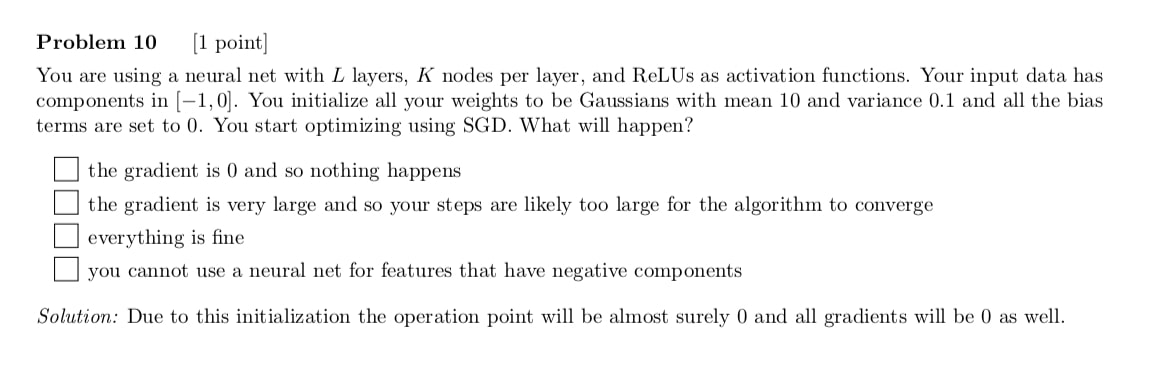### Problem 10 - Exam 2017 - Neural Net with ReLUs

Hello all !
I have trouble understanding the following question :First what is Gaussian weight ? aren't weights scalars ?

I would've set there's nothing wrong... Why is the gradient 0 ?

Gaussian means that they are normally distributed with N(10, 0.1), so each weight is a scalar but randomly initialised around the mean 10.

The ReLU has a zero gradient if the data is < 0 (you can inspect the graph visually), since we have no bias term the input is simply the dot product between the weight vector and the input vector. Because the input is between -1 and 0 (multiplied by something that will be about 10) the resulting dot product will be some negative scalar. The gradient for each activation function will thus be zero and the SGD algorithm will get stuck.

Page 1 of 1